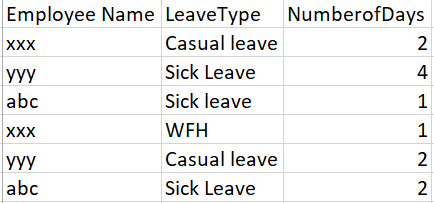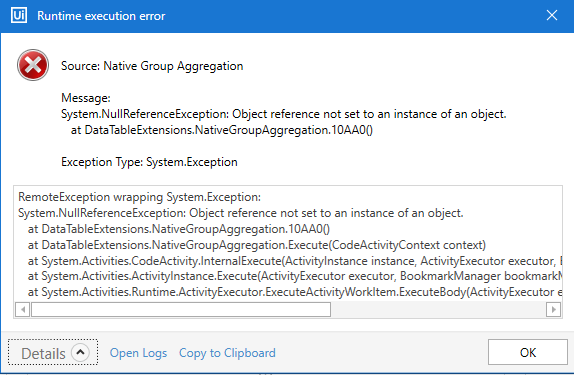# Sum values group by columns

Hi guys,
I have just started work with UIPath and have some issues.
select client_number, Currency, Start_Date, End_Date, sum(Amount) as sum_amount, sum(Commision) as commision
group by client_number, Currency, Start_Date, End_Date
The excel example is attached.
Thank you very much in advance!
DimaUntitled.xlsx (10.8 KB)

Hi @Stelz,

Refer this

Regards,
Arivu

2 Likes

HI @Stelz,

Regards
Balamurugan.S

3 Likes

Thank you very much. It works. Sorry for late reply

1 Like

How can you sum the values of a column without using balareva.datatable.activities ?

Hi @Fer
Try below code

``````DataTableName.Compute("Sum(Amount)", string.Empty);
``````

Regards,
Arivu3 Likes

Amount is the name of the column that i am searching values to sum?

Yes “Amount” is column name from the datatable which you want to sum

DataTableName is as System.Data.Datatable
sum = DataTableName.Compute(“Sum(Amount)”, string.Empty) where sum is defined as int32

Returns error

`sum= Convert.ToInt32(DataTableName. Compute("SUM(Amount)", string.Empty))`

Regards,
Arivu1 Like

Returns this error: Assign : Invalid usage of aggregate function Sum() and Type: Object.

Ok try below code

`DataTableName.AsEnumerable.Sum(Function(x) If(IsNumeric(x("Amount").ToString.Trim),CDbl(x("Amount").ToString.Trim),0))`

Regards,
Arivu2 Likes

How do you have declared the variable that is storing the code? Since I’m not getting AsEnumerable

1 Like

hi fellow, it seems this activity only can group and sum of on colunms, is it works while group multiple columns?

1 LikeHow do i get the total number of leaves for each leave type for each employee name ?

@Manjunath_Ramappa

Can you please post based on which column we have to do group by, and how should be the output.

Regards,
Mahesh

I need to get the output in terms of ‘total numberofDays’ for each employee Name for each ‘Leave type’
ex: xxx- casual leave - 2 (if there are multiple rows for casual leave for xxx, i should sum number of days)
Thanks in advanceIt is a late reply. But it helps others. The below thread will help to solve it.

Regards
Balamurugan.S